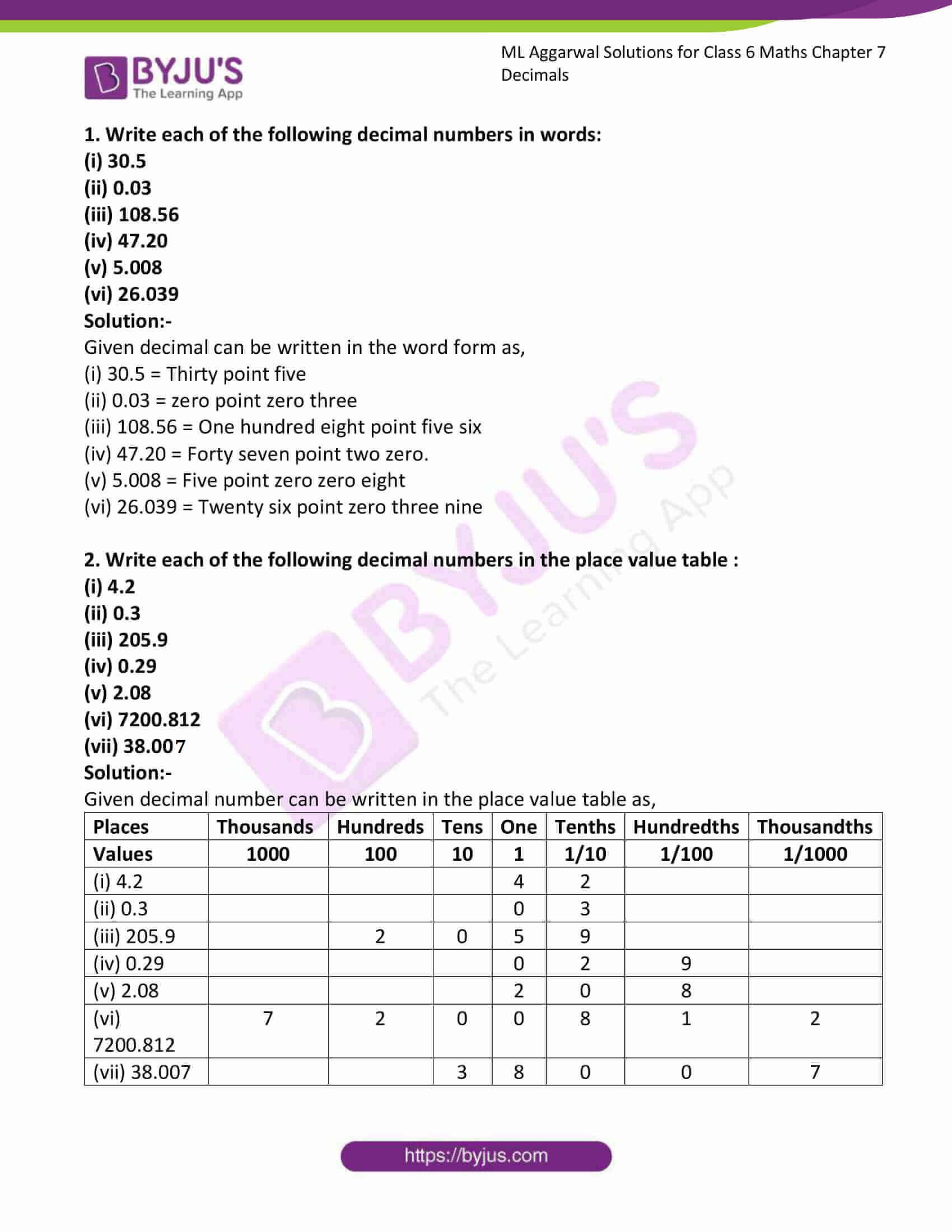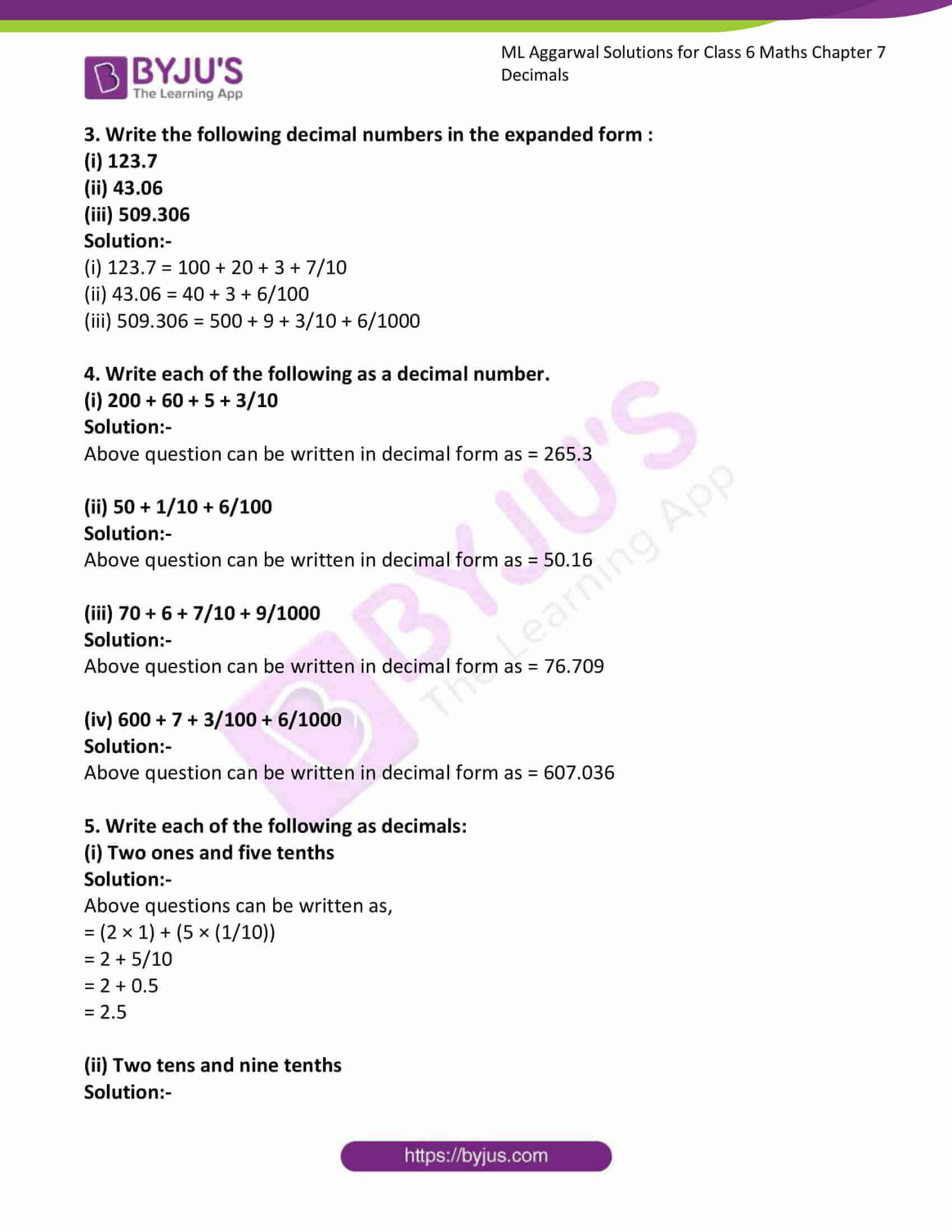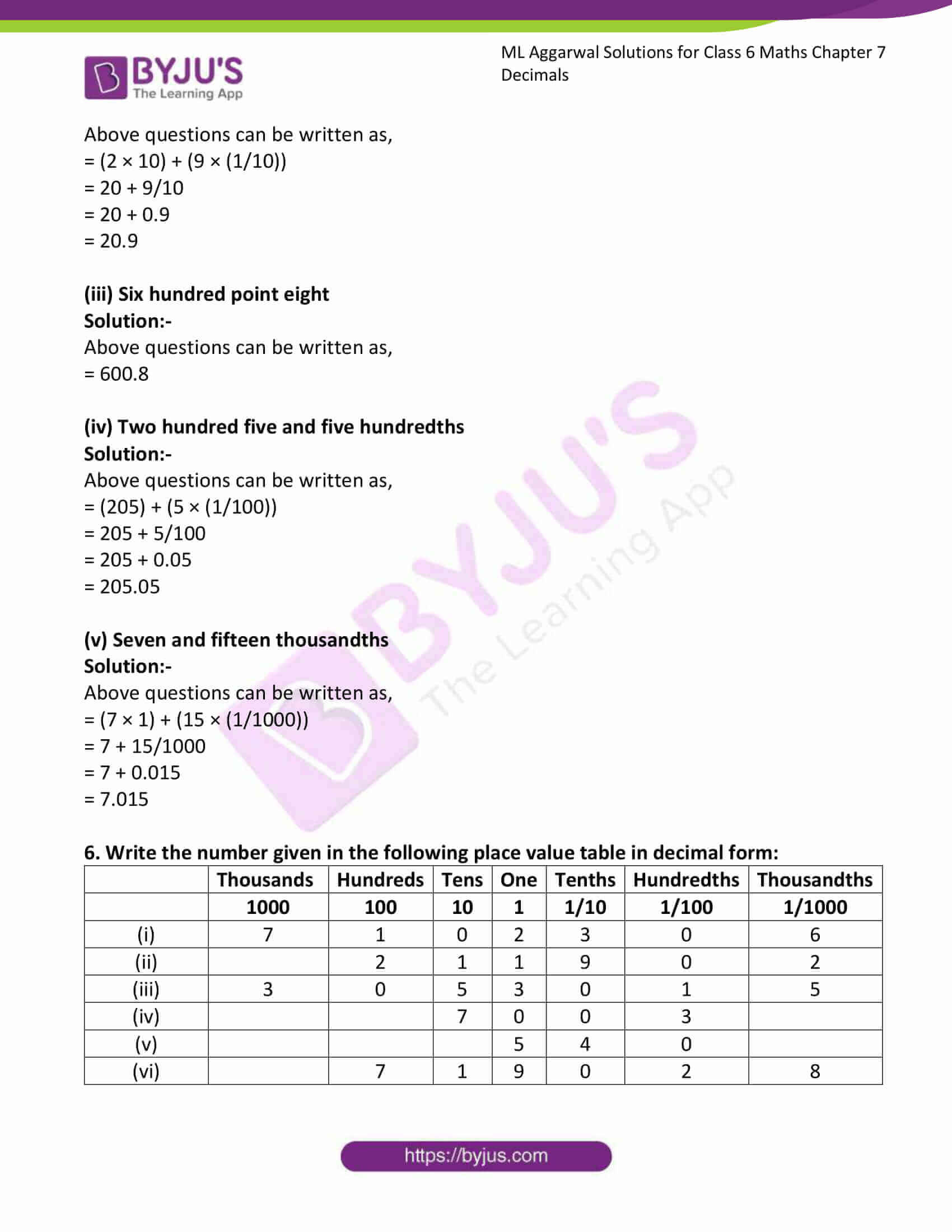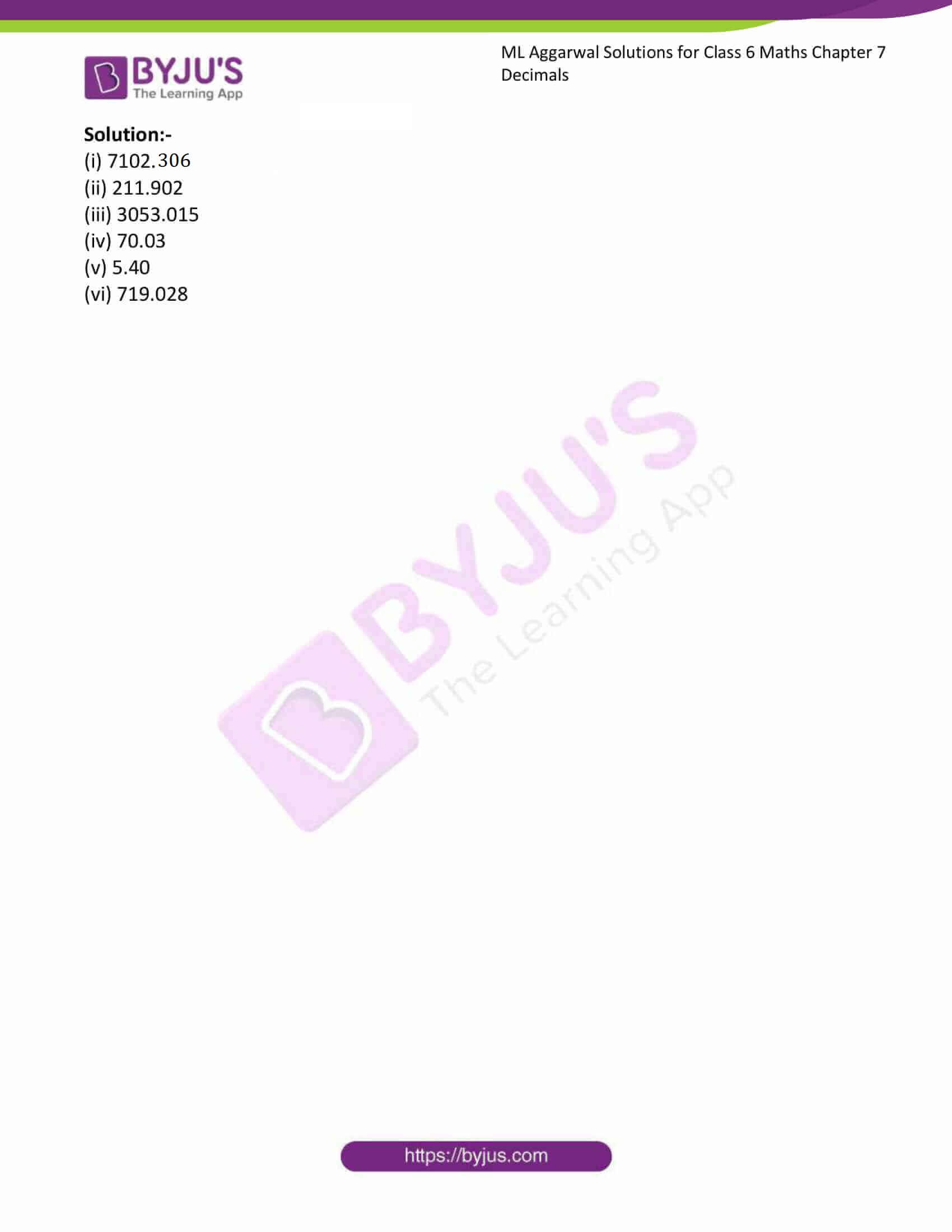# ML Aggarwal Solutions for Class 6 Maths Chapter 7 Decimals

ML Aggarwal Solutions for Class 6 Maths Chapter 7 Decimals is the most preferred study material due to its unique description of the concepts. In Chapter 7, a distinctive attempt is made to build an understanding of Decimals. Pursuing this ML Aggarwal Solutions for Class 6 Chapter 7 would ensure that you develop in-depth knowledge about Decimals.

Chapter 7 – Decimals is based on the study of any number from the base-ten number system. In this Chapter, we will be focusing on the numbers that contain one or more digits to the right of the point. The decimal point helps in separating the ones place from the tenths place in the number. Students can refer to ML Aggarwal Solutions for better understand of the concepts and can download the PDF available from the links given below.

## Download the PDF of ML Aggarwal Solutions for Class 6 Maths Chapter 7 Decimals### Access answers to ML Aggarwal Solutions for Class 6 Maths Chapter 7 Decimals

1. Write each of the following decimal numbers in words:
(i) 30.5
(ii) 0.03
(iii) 108.56
(iv) 47.20
(v) 5.008
(vi) 26.039

Solution:-

Given decimal can be written in the word form as,

(i) 30.5 = Thirty point five

(ii) 0.03 = zero point zero three

(iii) 108.56 = One hundred eight point five six

(iv) 47.20 = Forty seven point two zero.

(v) 5.008 = Five point zero zero eight

(vi) 26.039 = Twenty six point zero three nine

2. Write each of the following decimal numbers in the place value table :
(i) 4.2
(ii) 0.3
(iii) 205.9
(iv) 0.29
(v) 2.08
(vi) 7200.812
(vii) 38.007

Solution:-

Given decimal number can be written in the place value table as,

 Places Thousands Hundreds Tens One Tenths Hundredths Thousandths Values 1000 100 10 1 1/10 1/100 1/1000 (i) 4.2 4 2 (ii) 0.3 0 3 (iii) 205.9 2 0 5 9 (iv) 0.29 0 2 9 (v) 2.08 2 0 8 (vi) 7200.812 7 2 0 0 8 1 2 (vii) 38.007 3 8 0 0 7

3. Write the following decimal numbers in the expanded form :
(i) 123.7
(ii) 43.06
(iii) 509.306

Solution:-

(i) 123.7 = 100 + 20 + 3 + 7/10

(ii) 43.06 = 40 + 3 + 6/100

(iii) 509.306 = 500 + 9 + 3/10 + 6/1000

4. Write each of the following as a decimal number.

(i) 200 + 60 + 5 + 3/10

Solution:-

Above question can be written in decimal form as = 265.3

(ii) 50 + 1/10 + 6/100

Solution:-

Above question can be written in decimal form as = 50.16

(iii) 70 + 6 + 7/10 + 9/1000

Solution:-

Above question can be written in decimal form as = 76.709

(iv) 600 + 7 + 3/100 + 6/1000

Solution:-

Above question can be written in decimal form as = 607.036

5. Write each of the following as decimals:
(i) Two ones and five tenths

Solution:-

Above questions can be written as,

= (2 × 1) + (5 × (1/10))

= 2 + 5/10

= 2 + 0.5

= 2.5

(ii) Two tens and nine tenths

Solution:-

Above questions can be written as,

= (2 × 10) + (9 × (1/10))

= 20 + 9/10

= 20 + 0.9

= 20.9

(iii) Six hundred point eight

Solution:-

Above questions can be written as,

= 600.8

(iv) Two hundred five and five hundredths

Solution:-

Above questions can be written as,

= (205) + (5 × (1/100))

= 205 + 5/100

= 205 + 0.05

= 205.05

(v) Seven and fifteen thousandths

Solution:-

Above questions can be written as,

= (7 × 1) + (15 × (1/1000))

= 7 + 15/1000

= 7 + 0.015

= 7.015

6. Write the number given in the following place value table in decimal form:

 Thousands Hundreds Tens One Tenths Hundredths Thousandths 1000 100 10 1 1/10 1/100 1/1000 (i) 7 1 0 2 3 0 6 (ii) 2 1 1 9 0 2 (iii) 3 0 5 3 0 1 5 (iv) 7 0 0 3 (v) 5 4 0 (vi) 7 1 9 0 2 8

Solution:-

(i) 7102.306

(ii) 211.902

(iii) 3053.015

(iv) 70.03

(v) 5.40

(vi) 719.028